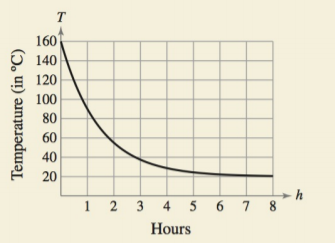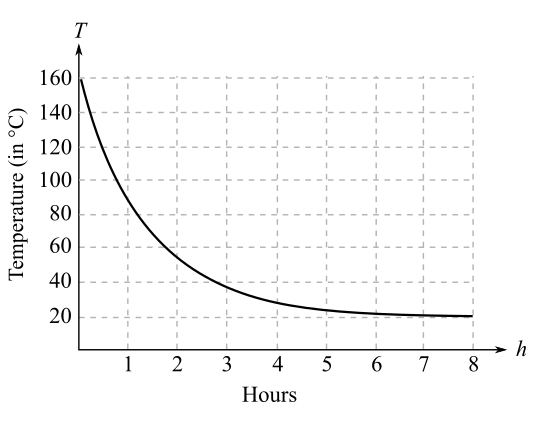Chapter 4.5, Problem 80E### Calculus: An Applied Approach (Min...

10th Edition
Ron Larson
ISBN: 9781305860919

#### Solutions

Chapter
Section### Calculus: An Applied Approach (Min...

10th Edition
Ron Larson
ISBN: 9781305860919
Textbook Problem
1 views

# HOW DO YOU SEE IT? The graph shows the temperature T ( in °C ) of an object h hours after it is removed from a furnace.(a) Find lim x → ∞ T . What does this limit represent?(b) When is the temperature changing most rapidly?

(a)

To determine

The value of limhT if the graph is showing the temperature T of a particular object after h hours of removing it from a furnace as below,Explanation

Given Information:

The graph showing the temperature T of a particular object after h hours of removing it from a furnace as below,

In the graph, the curve is declining when the number of hours are increasing which means that the temperature is decreasing as the number of hours are increasing.

The graph shows that the temperature is continuously decreasing from 160°C to

(b)

To determine

The time at which the temperature is changing most rapidly, if the graph shows the temperature T of a particular object after h hours of removing it from a furnace as below,### Still sussing out bartleby?

Check out a sample textbook solution.

See a sample solution

#### The Solution to Your Study Problems

Bartleby provides explanations to thousands of textbook problems written by our experts, many with advanced degrees!

Get Started

#### In the problem 1-4, solve the equations 3x14x9=57

Mathematical Applications for the Management, Life, and Social Sciences

#### . ∞ −∞ 1 −1

Study Guide for Stewart's Single Variable Calculus: Early Transcendentals, 8th

#### Using for |x| < 1,

Study Guide for Stewart's Multivariable Calculus, 8th